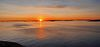# Patrik Jansson

More### Patrik Jansson

Professor of Computer Science

+46317725415

# Level-$$p$$-complexity of Boolean Functions: Using thinning, memoization, and polynomials

December 14, 2022

I gave a talk at the 2012-12 online meeting of IFIP WG 2.1 on Algorithmic Languages and Calculi. This is joint work (in progress) with Julia Jansson. The recording is available at YouTube and the slides here. The pre-print (submitted to JFP) is available on arXiv:2302.02473.

Abstract:

This talk presents a function for computing level-$$p$$-complexity of Boolean functions, and applies it to two-level iterated majority. The function is specified as a classical optimisation problem: first generate all candidates, then pick the best one. To make this function efficient we we use use thinning and memoization and for seperation of concerns we use type classes. The complexity is represented using polynomials, and the order relation used for thinning is implemented using polynomial factorisation and root-counting.

More details

Boolean functions are simply functions from $$n$$ bits to one bit, and they can describe digital circuits, voting systems, etc. An example of a Boolean function is majority, which returns the value that has majority among the $$n$$ input bits. The complexity of a Boolean function $$f$$ measures the cost of evaluating it: how much many bits of the input are needed to be certain about the result of $$f$$. There are many competing complexity measure but we focus on level-$$p$$-complexity --- a function of the probability that a bit is 1. The level-$$p$$-complexity $$D_p(f)$$ is the minimum expected cost when the input bits are independent and identically distributed with Bernoulli($$p$$) distribution. We specify the problem as choosing the minimum cost of all possible decision trees --- which directly translates to a clearly correct, but very efficient implementation. We then use thinning, memoisation, and symbolic comparison of polynomials to obtain an efficient implementation. Finally we compute the compute the complexity for two-level iterated majority and improve on an earlier result by J. Jansson.

Share